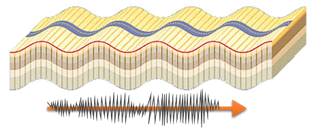# Static Seismic Analysis

### Seismic Standards

AutoPIPE includes the following standards for calculating seismic acceleration:

• A.L.A. 2002
• ASCE 2010
• China GB50011 2001
• Mexico CFE 2008
• User
• AutoPIPE handles up to 30 seismic acceleration vectors simultaneously. To facilitate the equations, the horizontal accelerations are combined with the vertical ones, so eight vectors are required to cover the most used vectors.

It is becoming more and more common to pre-fabricate modules at Skids and transport them to the job site for assembly. In this case, it is necessary to have four additional vectors to perform the transport acceleration calculation (No fluid in the pipes).

### Buried Piping

AutoPIPE Buried Model includes the pipe and its content mass, considering their density and therefore allowing many weight-related analyses like seismic, settlement, buoyancy, etc. Notice that other software change densities to zero for buried elements.

Seismic analysis can be performed in three ways:

• Static Seismic Acceleration can be applied to the model.
• Spectrum analysis can be performed.
• Seismic waves travel faster in the pipe than through the ground, AutoPIPE can apply a Thermal Equivalent of the wave propagation which makes it the preferred solution for studying pipelines.### Pipes in vertical structures

Seismic accelerations are calculated for the plant at ground level. For multi-level installations, simply multiply the basic design acceleration by the amplification factor of its height. This is because elevated structures have a greater seismic displacement as height increases. In general, seismic standards ask to multiply by:

$$F = 1 + \frac {2 z}{h}$$

Where z is the support height and h is the height of the structure. So F has a value ranging from 1 to 3.

In AutoPIPE it is very easy to apply this factor, since it is not necessary to modify the seismic accelerations, but rather select the pipes with the “Elevation Range” tool and apply the factor to each section.